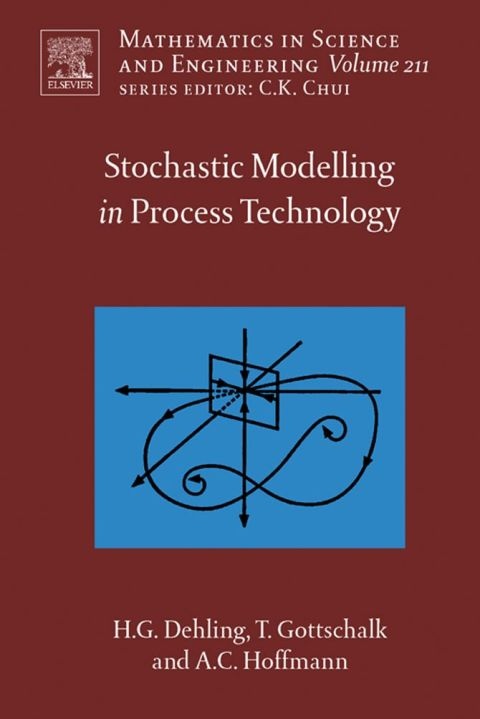• Author(s) Alex C. Timo; Hoffmann Herold G.; Gottschalk Dehling
• Edition211
• PublishedJuly 2007
• PublisherElsevier S & T
• ISBN9780444520265
There is an ever increasing need for modelling complex processes reliably. Computational modelling techniques, such as CFD and MD may be used as tools to study specific systems, but their emergence has not decreased the need for generic, analytical process models. Multiphase and multicomponent systems, and high-intensity processes displaying a highly complex behaviour are becoming omnipresent in the processing industry.
This book discusses an elegant, but little-known technique for formulating process models in process technology: stochastic process modelling.
The technique is based on computing the probability distribution for a single particle's position in the process vessel, and/or the particle's properties, as a function of time, rather than - as is traditionally done - basing the model on the formulation and solution of differential conservation equations.
Using this technique can greatly simplify the formulation of a model, and even make modelling possible for processes so complex that the traditional method is impracticable.
Stochastic modelling has sporadically been used in various branches of process technology under various names and guises. This book gives, as the first, an overview of this work, and shows how these techniques are similar in nature, and make use of the same basic mathematical tools and techniques.
The book also demonstrates how stochastic modelling may be implemented by describing example cases, and shows how a stochastic model may be formulated for a case, which cannot be described by formulating and solving differential balance equations.

Key Features:
- Introduction to stochastic process modelling as an alternative modelling technique
- Shows how stochastic modelling may be succesful where the traditional technique fails
- Overview of stochastic modelling in process technology in the research literature
- Illustration of the principle by a wide range of practical examples
- In-depth and self-contained discussions
- Points the way to both mathematical and technological research in a new, rewarding field

- Introduction to stochastic process modelling as an alternative modelling technique
- Shows how stochastic modelling may be succesful where the traditional technique fails
- Overview of stochastic modelling in process technology in the research literature
- Illustration of the principle by a wide range of practical examples
- In-depth and self-contained discussions
- Points the way to both mathematical and technological research in a new, rewarding field

# Stochastic Modelling in Process Technology

##### Get it instantly

Zookal account needed

\$153.88 \$157.30 Save \$3.42
• Author(s) Alex C. Timo; Hoffmann Herold G.; Gottschalk Dehling
• Edition211
• PublishedJuly 2007
• PublisherElsevier S & T
• ISBN9780444520265
There is an ever increasing need for modelling complex processes reliably. Computational modelling techniques, such as CFD and MD may be used as tools to study specific systems, but their emergence has not decreased the need for generic, analytical process models. Multiphase and multicomponent systems, and high-intensity processes displaying a highly complex behaviour are becoming omnipresent in the processing industry.
This book discusses an elegant, but little-known technique for formulating process models in process technology: stochastic process modelling.
The technique is based on computing the probability distribution for a single particle's position in the process vessel, and/or the particle's properties, as a function of time, rather than - as is traditionally done - basing the model on the formulation and solution of differential conservation equations.
Using this technique can greatly simplify the formulation of a model, and even make modelling possible for processes so complex that the traditional method is impracticable.
Stochastic modelling has sporadically been used in various branches of process technology under various names and guises. This book gives, as the first, an overview of this work, and shows how these techniques are similar in nature, and make use of the same basic mathematical tools and techniques.
The book also demonstrates how stochastic modelling may be implemented by describing example cases, and shows how a stochastic model may be formulated for a case, which cannot be described by formulating and solving differential balance equations.

Key Features:
- Introduction to stochastic process modelling as an alternative modelling technique
- Shows how stochastic modelling may be succesful where the traditional technique fails
- Overview of stochastic modelling in process technology in the research literature
- Illustration of the principle by a wide range of practical examples
- In-depth and self-contained discussions
- Points the way to both mathematical and technological research in a new, rewarding field

- Introduction to stochastic process modelling as an alternative modelling technique
- Shows how stochastic modelling may be succesful where the traditional technique fails
- Overview of stochastic modelling in process technology in the research literature
- Illustration of the principle by a wide range of practical examples
- In-depth and self-contained discussions
- Points the way to both mathematical and technological research in a new, rewarding field